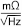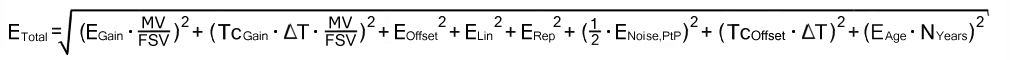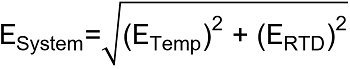# RTD/Pt1000 measurement

## RTD specification and conversion

Temperature measurement with a resistance-dependent RTD sensor generally consists of two steps:

• Electrical measurement of the resistance, if necessary in several ohmic measuring ranges
• Conversion (transformation) of the resistance into a temperature value by software means according to the set RTD type (Pt100, Pt1000…).

Both steps can take place locally in the Beckhoff measurement device. The transformation in the device can also be deactivated if it is to be calculated on a higher level in the control. Depending on the device type, several RTD conversions can be implemented which only differs in software. This means for Beckhoff RTD measurement devices that

• a specification table of the electrical resistance measurement is given
• and based on this, the effect for the temperature measurement is given below depending on the supported RTD type. Note that RTD characteristic curves are always realized as higher-order equations or by a sampling points table in the software, therefore a linear R→T transfer only makes sense in a narrow range.

## Application on the EPP3504‑0023

The EPP3504‑0023 supports the measurement of resistances up to 2 kΩ in 2/3/4‑wire measurement and the conversion of Pt1000 RTD sensors up to 2000 Ω / 266 °C.

Although the EPP3504‑0023 does not support a sole resistance measurement (without conversion to temperature), a resistance specification is given here because the temperature measurement is based on it.

## Note on 2-/3-/4-wire connection in R/RTD mode

With 2‑wire measurement, the line resistance of the sensor supply lines influences the measured value. If a reduction of this systematic error component is desirable for 2‑wire measurements, the resistance of the supply line to the measuring resistance should be taken into account, in which case the resistance of the supply line has to be determined first.

Taking into account the uncertainty associated with this supply line resistance, it can then be included statically in the calculation, in the EPP3504-0023 via CoE object 0x80n0:13.

Any change in resistance of the supply line due to ageing, for example, is not taken into account automatically. Just the temperature dependency of copper lines with approx. 4000 ppm/K (corresponds to 0.4%/K!) is not insignificant during 24/7 operation.

A 3‑wire measurement enables the systematic component to be eliminated, assuming that the two supply lines are identical. With this type of measurement, the lead resistance of a supply line is measured continuously. The value determined in this way is then deducted twice from the measurement result, thereby eliminating the line resistance. Technically, this leads to a significantly more reliable measurement. However, taking into account the measurement uncertainty, the gain from the 3‑wire connection is less significant, since this assumption is subject to high uncertainty, in view of the fact that the individual line that was not measured may be damaged, or a varying resistance may have gone unnoticed.

Therefore, although technically the 3‑wire connection is a tried and tested approach, for measurements that are methodological assessed based on measurement uncertainty, we strongly recommend fully‑compensated 4‑wire connection.

With both 2‑wire and 3‑wire connection, the contact resistances of the terminal contacts influence the measuring process. The measuring accuracy can be further increased by a user‑side adjustment with the signal connection plugged in.

 Note Measurement of small resistancesEspecially for measurements in the range < 10 Ω, the 4‑wire connection is absolutely necessary due to the relatively high supply and contact resistances. It should also be considered that with such low resistances the relative measurement error in relation to the full scale value (FSV) can become high ‑ for such measurements resistance measurement terminals with small measuring ranges such as EL3692 in 4‑wire measurement should be used if necessary.

Corresponding considerations also lead to the common connection methods in bridge operation:

• Full bridge: 4‑wire connection without line compensation, 6‑wire connection with full line compensation
• Half bridge: 3‑wire connection without line compensation, 5‑wire connection with full line compensation
• Quarter bridge: 2‑wire connection without line compensation, 3‑wire connection with theoretical line compensation and 4‑wire connection with full line compensation

Resistance measurement 2kΩ

2/3-wire 1)

4-wire

Operation mode

3 V feed voltage fixed setting on +Uv
internal 1 kΩ reference resistance at –I2
Supply current is given by:
3 V / (1 kΩ + Rmeasurement) → max. 3 mA

Measuring range, nominal

2 kΩ (corresponds to PT1000 +266°C)

Measuring range, end value (FSV)

2 kΩ

Measuring range, technically usable

0 … 2 kΩ

PDO LSB (Extended Range)

Extended range is not supported for resistance measurement

PDO LSB (Legacy Range)

Resistance measurement not available as separate measuring range on EPP3504-0023.

## Preliminary specifications:

Resistance measurement 2kΩ

2/3-wire 1)

4-wire

Basic accuracy: Measuring deviation at 23°C, with averaging, with Offset , typ.

< ± 0.024 %FSV
< ± 240 ppmFSV
< ± 480 mΩ

< ± 0.022 %FSV
< ± 220 ppmFSV
< ± 440 mΩ

Offset/Zero point deviation (at 23°C)

EOffset

< 80 ppmFSV

< 60 ppmFSV

Gain/scale/amplification deviation (at 23°C)

EGain

< 180 ppm

< 160 ppm

Non-linearity over the whole measuring range

ELin

< 130 ppmFSV

< 130 ppmFSV

Repeatability (at 23°C)

ERep

< 20 ppmFSV

< 20 ppmFSV

Noise (without filtering, at 23°C)

ENoise, PtP

< 220.0 ppmFSV
< 1719 digits
< 440.0 mΩ

< 220.0 ppmFSV
< 1719 digits
< 440.0 mΩ

ENoise, RMS

< 37.0 ppmFSV
< 289 digits
< 74.0 mΩ

< 37.0 ppmFSV
< 289 digits
< 74.0 mΩ

Max. SNR

> 88.6 dB

> 88.6 dB

Noisedensity@1kHz

< 1.05< 1.05Noise (with 50 Hz FIR filter, at 23°C)

ENoise, PtP

< 14.0 ppmFSV
< 109 digits
< 28.0 mΩ

< 14.0 ppmFSV
< 109 digits
< 28.0 mΩ

ENoise, RMS

< 2.3 ppmFSV
< 18 digits
< 4.6 mΩ

< 2.3 ppmFSV
< 18 digits
< 4.6 mΩ

Max. SNR

> 112.8 dB

> 112.8 dB

Common-mode rejection ratio (without filter) 3)

tbd.

tbd.

Common-mode rejection ratio (with 50 Hz FIR filter) 3)

tbd.

tbd.

Temperature coefficient, typ.

TcGain

< 20 ppm/K

< 20 ppm/K

TcOffset

< 8 ppmFSV/K
< 16 mΩ/K

< 3 ppmFSV/K
< 6 mΩ/K

Largest short-term deviation during a specified electrical interference test, typ.

tbd. %FSV

tbd. %FSV

1) The offset specification does not apply to 2-wire operation, since the offset is increased on the device side. Therefore, a system-side offset adjustment is recommended, see "Note on 2-/3-/4-wire connection in R/RTD mode". The final targeting basic acuuracy within the 2-wire operation is mainingly dependent by the quality of this system-side offset adjustment.

3) Values related to a common mode interference between SGND and internal ground.

## RTD measuring range

In temperature mode, only the legacy range is available, the extended range is not available.

The temperature display in [°C/digit] (e.g. 0.1°/digit or 0.01°/digit) is independent from the electrical measurement. It is “just” a display setting and results from the PDO setting, see chapter "Comissioning".Data for the sensor types in the following tableThe values for the sensor types listed in the following table are shown here merely for informative purposes as an orientation aid. All data are given without guarantee and must be cross-checked against the data sheet for the respective sensor employed.

The RTD measurement consists of a chain of measuring and computing elements that affect the attainable measurement deviation:

The given resistance specification is decisive for the attainable temperature measurement accuracy. It is applied to the possible RTD types in the following.

On account of

• the non-linearity existing in the RTD and thus the high dependency of the specification data on the sensor temperature Tsens and
• the influence of the ambient temperature on the analog input device employed (leads to a change in Tmeasured on account of ∆Tambient although Tsens = constant)

no detailed temperature specification table is given in the following, but

• a short table specifying the electrical measuring range and orientation value for the basic accuracy
• a graph of the basic accuracy over Tsens (this at two example ambient temperatures so that the attainable basic accuracy is implied on account of the actual existing ambient temperature)
• equations for calculating further parameters (offset/gain/non-linearity/repeatability/noise) if necessary from the resistance specification at the desired operating point

RTD types supported by the EPP3504-0023:

• Pt1000 according to DIN EN 60751/IEC751 with α= 0.0039083 [1/C°]

RTD temperature measurement

Pt1000 2-wire

Pt1000 3-wire

Pt1000 4-wire

Electrical measuring range used

2 kΩ

Starting value

-200°C ≈ 185.2 Ω

End value

266°C ≈ 2000 Ω

PDO LSB (legacy range only)

0.1/0.01/0.001°C/digit, depending on PDO setting

Basic accuracy: measurement deviation at 23 °C ambient temperature, with averaging, typ.

The achievable measurement uncertainty, as a system side offset, is essentially dependent on the line resistances and can at best reach the value of the 3-wire measurement.

< ±132 mK

(preliminary value)

< ±120 mK

(preliminary value)

Temperature coefficient 2), typ.

< 6.6 mK/K

(preliminary value)

< 5.4 mK/K

(preliminary value)

2) The temperature coefficient, i.e. the change in the measured temperature value in relation to the change in the ambient temperature of the box module, is not constant, as can be seen in the following plot. The value at a sensor temperature of 0 °C is given here as an orientation value. Further values can be taken from the plot.

## Basic accuracy for Pt1000, 4-wire connection:

If further specification data are of interest, they can or must be calculated from the values given in the resistance specification.

The sequence:

• General: The conversion is explained here only for one measuring point (a certain input signal); the steps simply must be repeated in case of several measuring points (up to the entire measuring range).
• If the measured resistance at the measured temperature measuring point is unknown, the measured value (MW) in [Ω] must be determined:
MW = RMeasuring point (TMeasuring point) with the help of an R→T table
• The deviation at this resistance value is calculated
• Via the total equation• or a single value, e.g. ESingle = 15 ppmFSV
• the measurement uncertainty in [Ω] must be calculated:
EResistance(RMeasuring point) = ETotal(RMeasuring point) ⋅ FSV
or: EResistance(RMeasuring point) = ESingle(RMeasuring point) ⋅ FSV
or (if already known) e.g.: EResistance(RMeasuring point) = 0.03 Ω
• The slope at the point used must then be determined:
ΔRproK(TMeasuring point) = [ R(TMeasuring point + 1 °C) – R(TMeasuring point )] / 1 °C
with the help of an R→T table
• The temperature measurement uncertainty can be calculated from the resistance measurement uncertainty and the slope
ETemp(RMeasuring point) = (EResistance(TMeasuring point)) / (ΔRproK(TMeasuring point) )
• To determine the error of the entire system consisting of RTD and the measuring device in [°C], the two errors must be added together quadratically:The numerical values used in the following three examples are for illustration purposes. The specification values given in the technical data remain authoritative.

## Example 1:

Basic accuracy of an ELM3504 at 35 °C ambient temperature, measurement of -100 °C in the PT1000 interface (4-wire), without the influence of noise and aging:

TMeasuring point = -100 °C

MW = RPT1000, -100 °C = 602.56 Ω

= 86.238 ppmFSV

EResistance(RMeasuring point) = 86.238 ppmFSV ⋅ 2000 Ω = 0.1725 Ω

ΔRproK(TMeasuring point) = (R(-99 °C) – R(-100 °C)) / (1 °C) = 4.05 Ω/°C

EELM3504@35°C, PT1000, -100 °C = (0.1725 Ω)/(4.05 Ω/°C) ≈ 0.043 °C (means ±0.043 °C)

## Example 2:

Consideration of the repeatability alone under the above conditions:

TMeasuring point = -100 °C

MW = RMeasuring point (-100 °C) = 602.56 Ω

ESingle = 10 ppmFSV

EResistance= 10 ppmFSV ⋅ 2000 Ω = 0.02 Ω

ΔRproK(TMeasuring point) = (R-99 °C – R-100 °C) / 1 °C = 4.05 Ω/°C

ETemp(RMeasuring point) = 0.02 Ω / 4.05 Ω/°C ≈ 0.005 °C (means ±0.005 °C)

## Example 3:

Consideration of the RMS noise alone without filter under the above conditions:

TMeasuring point = -100 °C

MW = RMeasuring point (-100 °C) = 602.56 Ω

ESingle = 37 ppmFSV

EResistance= 37 ppmFSV ⋅ 2000 Ω = 0.074 Ω

ΔRproK(TMeasuring point) = (R-99 °C – R-100 °C) / 1 °C = 4.05 Ω/°C

ETemp(RMeasuring point) = 0.074 Ω / 4.05 Ω/°C ≈ 0.018 °C (means ± 0.018 °C)

## Example 4:

If the noise ENoise, PtP of the above example terminal is considered not for one sensor point -100 °C but in general, the following plot results: